# Motion in two dimensions

## Two dimensional Motion

• Two Dimensional Motion is the motion of the particle in a fixed plane. Unlike rectilinear motion where particle moves in a straight line,here particles moves along in the x-y plane. Both x and y coordinates of the particles changes with time
• Examples of Motion in Two dimensions are Projectile motion(Motion of the football ) ,Circular Motion( earth motion around the sun), Motion of a billiard ball on the billiard table, Motion of the boat etc
• It can be uniformly acclerated motion or non-uniformly accelerated motion
• Now lets understand position vector, average velocity, Instantaneous velocity,Average and instantaneous acceleration pertaining to motion in two dimensions

## Position vector & Displacement

In the coordinate system, the line joining the origin O to the point P in the system is called the position vector of Point P
$\vec{OP}= x\hat{i} + y\hat{j} + z\hat{k}$

Displacement is the Vector joining two points in the Coordinate system
Let Point P and Q are there
Position Vector of Point P
$\vec{OP}= x_1 \hat{i} + y_1 \hat{j} + z_1 \hat{k}$
Position Vector of Point Q
$\vec{OQ}= x_2 \hat{i} + y_2 \hat{j} + z_2 \hat{k}$
Then Vector $\vec{PQ}$ is defined as
$\vec{PQ}=\vec{OQ} - \vec{OP}$
$=(x_2 -x_1) \hat{i} + (y_2 -y_1)\hat{j} + (z_2 -z_1)\hat{ k}$

### Concept Map's for Position and displacement in two dimensional motion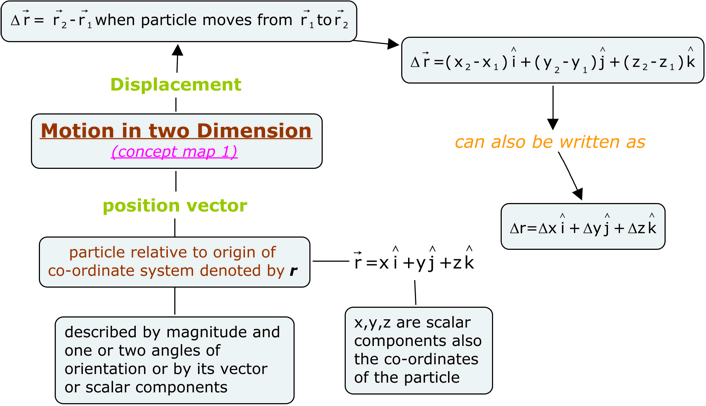## Average velocity

• Consider a particle moving along a curved path in x-y plane shown below in the figure
• Suppose at any time,particle is at the point P and after some time 't' is at point Q where points P and Q represents the position of particle at two different points.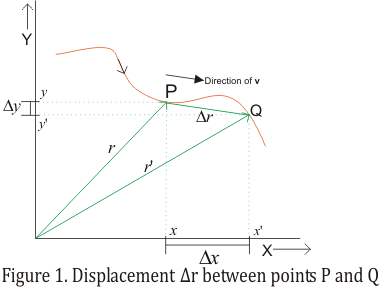• Position of particle at point P is described by the Position vector r from origin O to P given by
r=xi+yj
where x and y are components of r along x and y axis
• As particle moves from P to Q,its displacement would be would be Δr which is equal to the difference in position vectors r and r'.Thus
Δr = r'-r = (x'i+y'j)-(xi+yj) = (x'-x)i+(y'-y)j = Δxi+Δyj                                          (1)
where Δx=(x'-x) and Δy=(y'-y)
• If Δt is the time interval during which the particle moves from point P to Q along the curved path then average velocity(vavg) of particle is the ratio of displacement and corresponding time interval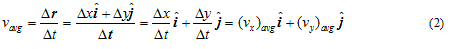since vavgr/Δt , the direction of average velocity is same as that of Δr
• Magnitude of Δr is always the straight line distance from P to Q regardless of any shape of actual path taken by the particle.
• Hence average velocity of particle from point P to Q in time interval Δt would be same for any path taken by the particle.

## Instantaneous velocity

• We already know that instantaneous velocity is the velocity of the particle at any instant of time or at any point of its path.
• If we bring point Q more and more closer to point P and then calculate average velocity over such a short displacement and time interval then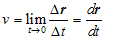where v is known as the instantaneous velocity of the particle.
• Thus, instantaneous velocity is the limiting value of average velocity as the time interval approaches zero.
• As the point Q approaches P, direction of vector Δr changes and approaches to the direction of the tangent to the path at point P. So instantaneous vector at any point is tangent to the path at that point.
• Figure below shows the direction of instantaneous velocity at point P.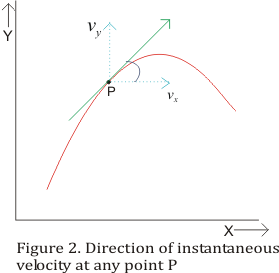• Thus, direction of instantaneous velocity v at any point is always tangent to the path of particle at that point.
• Like average velocity we can also express instantaneous velocity in component form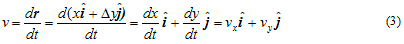where vx and vy are x and y components of instantaneous velocity.
• Magnitude of instantaneous velocity is
$|\vec {v}|= \sqrt {(v_x^2+ v_y^2)}$
and angle θ which velocity vector makes with x-axis is
$\tan \theta= \frac {v_y}{v_x}$
• Expression for instantaneous velocity is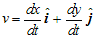Thus, if expression for the co-ordinates x and y are known as function of time then we can use equations derived above to find x and y components of velocity.

## Average and instantaneous acceleration

• Suppose a particle moves from point P to point Q in x-y plane as shown below in the figure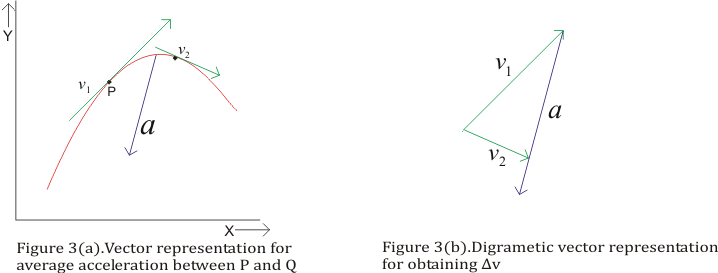• Suppose v1 is the velocity of the particle at point P and v2 is the velocity of particle at point Q
• Average acceleration is the change in velocity of particle from v1 to v2 in time interval Δt as particle moves from point P to Q. Thus average acceleration is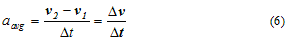Average acceleration is the vector quantity having direction same as that of Δv.
• Again if point Q approaches point P, then limiting value of average acceleration as time approaches zero defines instantaneous acceleration or simply the acceleration of particle at that point. This, instantaneous acceleration is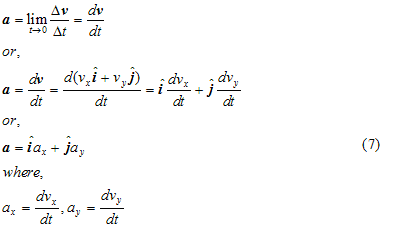• Figure below shows instantaneous acceleration a at point P.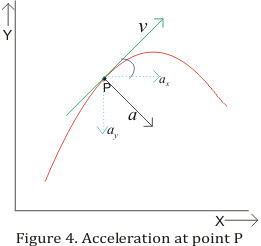• Instantaneous acceleration does not have same direction as that of velocity vector instead it must lie on the concave side of the curved surface.
• Thus velocity and acceleration vectors may have any angle between 0 to 180 degree between them.

## Concept Map's for Velocity and acceleration in two dimensional motion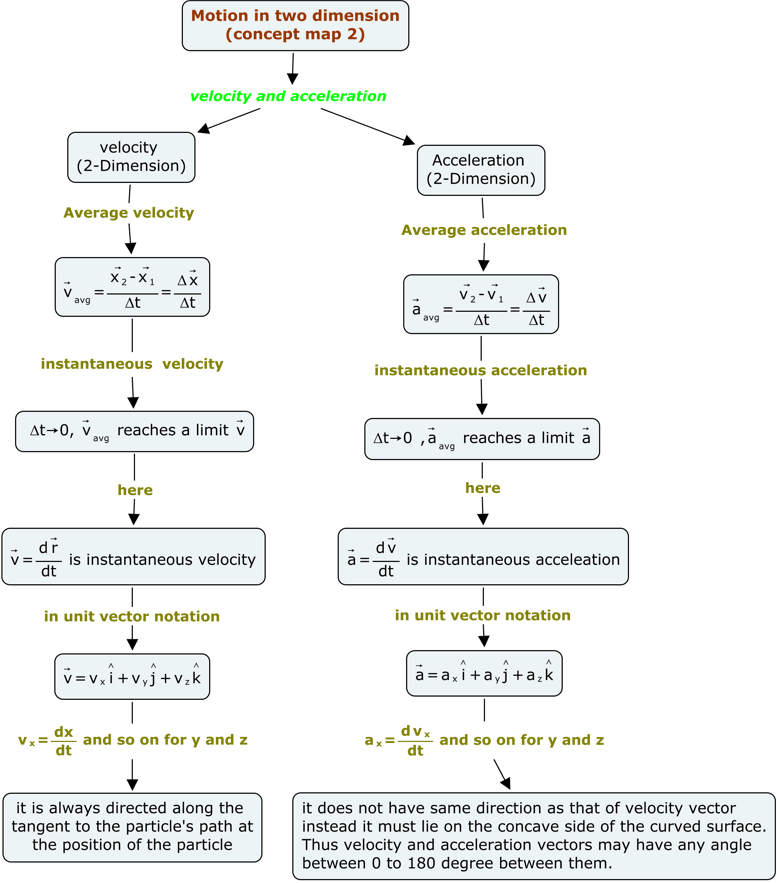## Solved examples of Motion in a plane

Question
The position of a object in a two dimensional motion is given by
$\vec{r}= 3t \hat{i} + 2t^2 \hat {j}$
Where t is in second and coefficients have the proper units for r to be in centimetres
a) Find $\vec{v(t)}$ and $\vec{a(t)}$ of the object
b) Find the magnitude and direction of the velocity at t=3 sec
Solution
It is given in the questions
$\vec{r}= 3t \hat{i} + 2t^2 \hat {j}$
Now
$\vec{v(t)} = \frac {d \vec{r}}{dt}$
Therefore,
$\vec{r}= 3t \hat{i} + 2t^2 \hat {j}$
$\vec{r} =\frac {d[3t \hat{i} + 2t^2 \hat {j}]}{dt} =3\hat{i} +4t\hat{j}$
Now
$\vec {a(t)} = \frac {d \vec{v}}{dt}$
Therefore,
$\vec {a(t)} =\frac {d[3\hat{i} +4t\hat{j}]}{dt} =4\hat{j}$
So acceleration is 4 cm/s2 across y-axis

Now velocity at 3 sec

$\vec{v(t)}=3\hat{i} +4t\hat{j}=3\hat{i} +12\hat{j}$
So its magnitude is $\sqrt {(3^2 +12^2)} = 12.4$ cm/s
And direction will be $tan^{-1} \frac {v_y}{v_x} =tan^{-1} (4) =76^0$